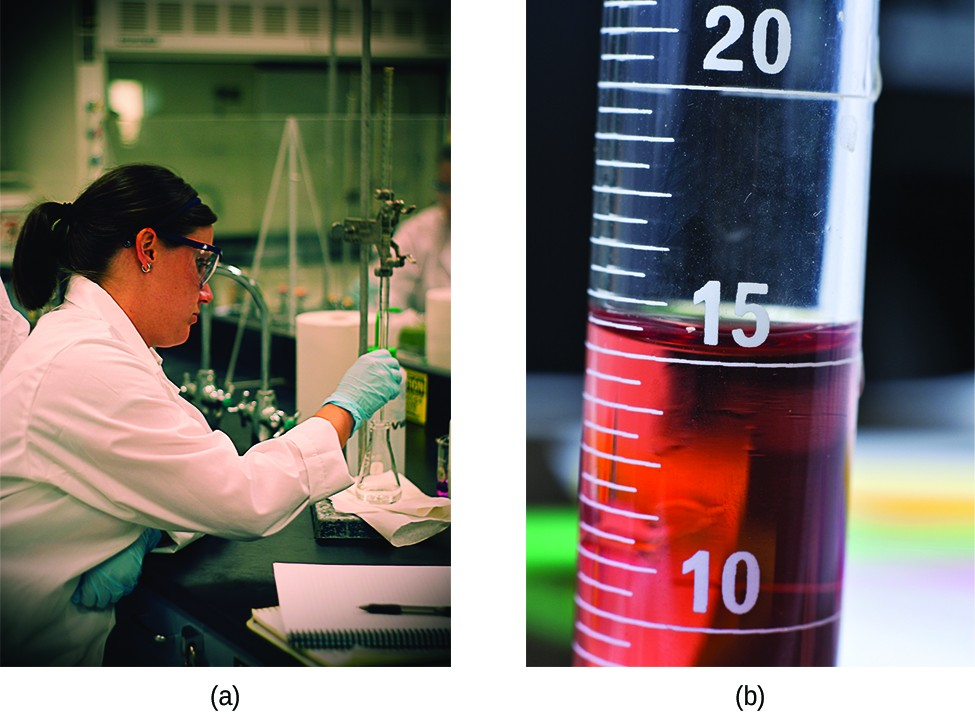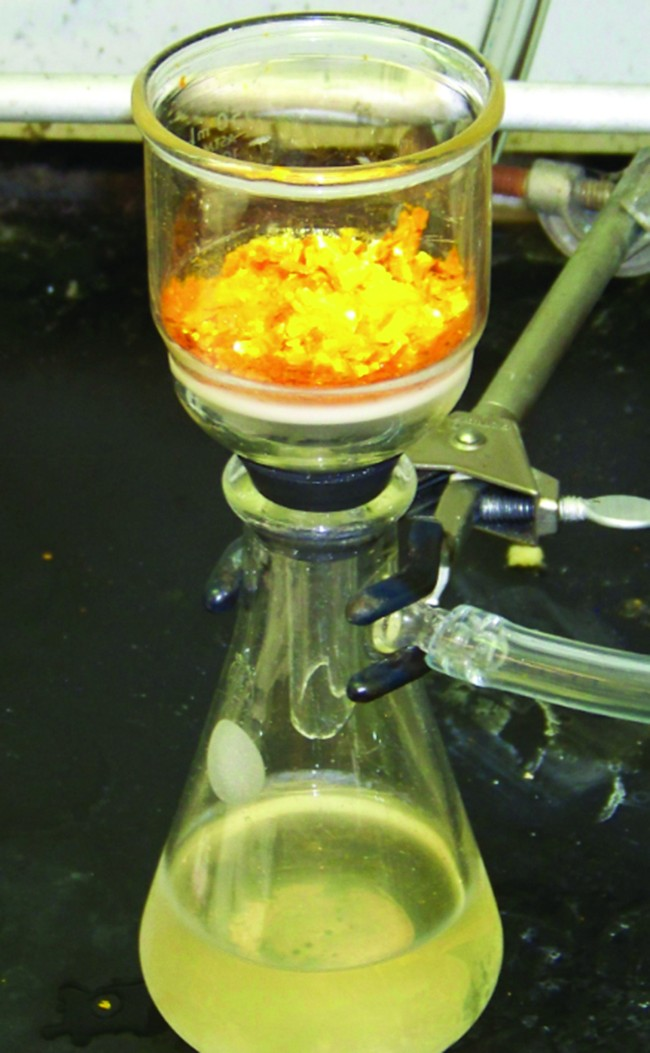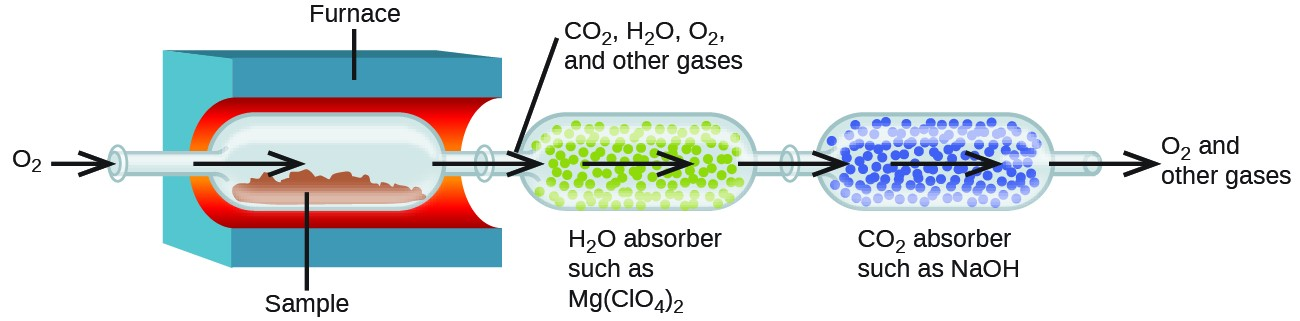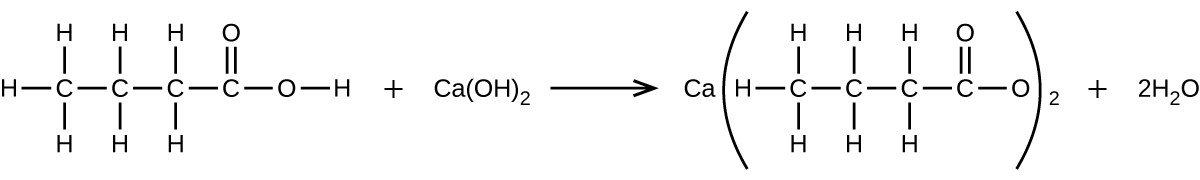## Quantitative Chemical Analysis

### Learning Objectives

By the end of this section, you will be able to:

• Describe the fundamental aspects of titrations and gravimetric analysis.
• Perform stoichiometric calculations using typical titration and gravimetric data.

In the eighteenth century, the strength (actually the concentration) of vinegar samples was determined by noting the amount of potassium carbonate, K2CO3, which had to be added, a little at a time, before bubbling ceased. The greater the weight of potassium carbonate added to reach the point where the bubbling ended, the more concentrated the vinegar.

We now know that the effervescence that occurred during this process was due to reaction with acetic acid, CH3CO2H, the compound primarily responsible for the odor and taste of vinegar. Acetic acid reacts with potassium carbonate according to the following equation:

$2{\text{CH}}_{3}{\text{CO}}_{2}\text{H}\text{(}aq\text{)}+{\text{K}}_{2}{\text{CO}}_{3}\text{(}s\text{)}\rightarrow{\text{KCH}}_{3}{\text{CO}}_{3}\text{(}aq\text{)}+{\text{CO}}_{2}\text{(}g\text{)}+{\text{H}}_{2}\text{O}\text{(}l\text{)}\text{.}$

The bubbling was due to the production of CO2.

The test of vinegar with potassium carbonate is one type of quantitative analysis—the determination of the amount or concentration of a substance in a sample. In the analysis of vinegar, the concentration of the solute (acetic acid) was determined from the amount of reactant that combined with the solute present in a known volume of the solution. In other types of chemical analyses, the amount of a substance present in a sample is determined by measuring the amount of product that results.

## TitrationFigure 1. (a) A student fills a buret in preparation for a titration analysis. (b) A typical buret permits volume measurements to the nearest 0.01 mL. (credit a: modification of work by Mark Blaser and Matt Evans; credit b: modification of work by Mak Blaser and Matt Evans)

The described approach to measuring vinegar strength was an early version of the analytical technique known as titration analysis. A typical titration analysis involves the use of a buret (Figure 1) to make incremental additions of a solution containing a known concentration of some substance (the titrant) to a sample solution containing the substance whose concentration is to be measured (the analyte). The titrant and analyte undergo a chemical reaction of known stoichiometry, and so measuring the volume of titrant solution required for complete reaction with the analyte (the equivalence point of the titration) allows calculation of the analyte concentration.

The equivalence point of a titration may be detected visually if a distinct change in the appearance of the sample solution accompanies the completion of the reaction. The halt of bubble formation in the classic vinegar analysis is one such example, though, more commonly, special dyes called indicators are added to the sample solutions to impart a change in color at or very near the equivalence point of the titration.

Equivalence points may also be detected by measuring some solution property that changes in a predictable way during the course of the titration. Regardless of the approach taken to detect a titration’s equivalence point, the volume of titrant actually measured is called the end point. Properly designed titration methods typically ensure that the difference between the equivalence and end points is negligible. Though any type of chemical reaction may serve as the basis for a titration analysis, the three described in this chapter (precipitation, acid-base, and redox) are most common. Additional details regarding titration analysis are provided in the chapter on acid-base equilibria.

### Example 1: Titration Analysis

The end point in a titration of a 50.00-mL sample of aqueous HCl was reached by addition of 35.23 mL of 0.250 M NaOH titrant. The titration reaction is:

$\text{HCl}\text{(}aq\text{)}+\text{NaOH}\text{(}aq\text{)}\rightarrow\text{NaCl}\text{(}aq\text{)}+{\text{H}}_{2}\text{O}\text{(}l\text{)}\text{.}$

What is the molarity of the HCl?

A 20.00-mL sample of aqueous oxalic acid, H2C2O4, was titrated with a 0.09113-M solution of potassium permanganate.

$2{\text{MnO}}_{4}{}^{-}\text{(}aq\text{)}+5{\text{H}}_{2}{\text{C}}_{2}{\text{O}}_{4}\text{(}aq\text{)}+6{\text{H}}^{+}\text{(}aq\text{)}\rightarrow 10{\text{CO}}_{2}\text{(}g\text{)}+2{\text{Mn}}^{\text{2+}}\text{(}aq\text{)}+8{\text{H}}_{2}\text{O}\text{(}l\text{)}$

A volume of 23.24 mL was required to reach the end point. What is the oxalic acid molarity?

## Gravimetric AnalysisFigure 2. Precipitate may be removed from a reaction mixture by filtration.

A gravimetric analysis is one in which a sample is subjected to some treatment that causes a change in the physical state of the analyte that permits its separation from the other components of the sample. Mass measurements of the sample, the isolated analyte, or some other component of the analysis system, used along with the known stoichiometry of the compounds involved, permit calculation of the analyte concentration. Gravimetric methods were the first techniques used for quantitative chemical analysis, and they remain important tools in the modern chemistry laboratory.

The required change of state in a gravimetric analysis may be achieved by various physical and chemical processes. For example, the moisture (water) content of a sample is routinely determined by measuring the mass of a sample before and after it is subjected to a controlled heating process that evaporates the water.

Also common are gravimetric techniques in which the analyte is subjected to a precipitation reaction of the sort described earlier in this chapter. The precipitate is typically isolated from the reaction mixture by filtration, carefully dried, and then weighed (Figure 2). The mass of the precipitate may then be used, along with relevant stoichiometric relationships, to calculate analyte concentration.

### Example 2: Gravimetric Analysis

A 0.4550-g solid mixture containing CaSO4 is dissolved in water and treated with an excess of Ba(NO3)2, resulting in the precipitation of 0.6168 g of BaSO4.

${\text{CaSO}}_{4}\text{(}aq\text{)}+\text{Ba}{\text{(}{\text{NO}}_{3}\text{)}}_{2}\text{(}aq\text{)}\rightarrow{\text{BaSO}}_{4}\text{(}s\text{)}+\text{Ca}{\text{(}{\text{NO}}_{3}\text{)}}_{2}\text{(}aq\text{)}$

What is the concentration (percent) of CaSO4 in the mixture?

What is the percent of chloride ion in a sample if 1.1324 g of the sample produces 1.0881 g of AgCl when treated with excess Ag+?

${\text{Ag}}^{+}\text{(}aq\text{)}+{\text{Cl}}^{-}\text{(}aq\text{)}\rightarrow\text{AgCl}\text{(}s\text{)}$

The elemental composition of hydrocarbons and related compounds may be determined via a gravimetric method known as combustion analysis. In a combustion analysis, a weighed sample of the compound is heated to a high temperature under a stream of oxygen gas, resulting in its complete combustion to yield gaseous products of known identities. The complete combustion of hydrocarbons, for example, will yield carbon dioxide and water as the only products. The gaseous combustion products are swept through separate, preweighed collection devices containing compounds that selectively absorb each product (Figure 3). The mass increase of each device corresponds to the mass of the absorbed product and may be used in an appropriate stoichiometric calculation to derive the mass of the relevant element.Figure 3. This schematic diagram illustrates the basic components of a combustion analysis device for determining the carbon and hydrogen content of a sample.

### Example 3: Combustion Analysis

Polyethylene is a hydrocarbon polymer used to produce food-storage bags and many other flexible plastic items. A combustion analysis of a 0.00126-g sample of polyethylene yields 0.00394 g of CO2 and 0.00161 g of H2O. What is the empirical formula of polyethylene?

A 0.00215-g sample of polystyrene, a polymer composed of carbon and hydrogen, produced 0.00726 g of CO2 and 0.00148 g of H2O in a combustion analysis. What is the empirical formula for polystyrene?

### Key Concepts and Summary

The stoichiometry of chemical reactions may serve as the basis for quantitative chemical analysis methods. Titrations involve measuring the volume of a titrant solution required to completely react with a sample solution. This volume is then used to calculate the concentration of analyte in the sample using the stoichiometry of the titration reaction. Gravimetric analysis involves separating the analyte from the sample by a physical or chemical process, determining its mass, and then calculating its concentration in the sample based on the stoichiometry of the relevant process. Combustion analysis is a gravimetric method used to determine the elemental composition of a compound by collecting and weighing the gaseous products of its combustion.

### Exercises

1. What volume of 0.0105-M HBr solution is be required to titrate 125 mL of a 0.0100-M Ca(OH)2 solution?$\text{Ca}{\text{(}\text{OH}\text{)}}_{2}\text{(}aq\text{)}+2\text{HBr}\text{(}aq\text{)}\rightarrow{\text{CaBr}}_{2}\text{(}aq\text{)}+2{\text{H}}_{2}\text{O}\text{(}l\text{)}$
2. Titration of a 20.0-mL sample of acid rain required 1.7 mL of 0.0811 M NaOH to reach the end point. If we assume that the acidity of the rain is due to the presence of sulfuric acid, what was the concentration of sulfuric acid in this sample of rain?
3. What is the concentration of NaCl in a solution if titration of 15.00 mL of the solution with 0.2503 M AgNO3 requires 20.22 mL of the AgNO3 solution to reach the end point? ${\text{AgNO}}_{3}\text{(}aq\text{)}+\text{NaCl}\text{(}aq\text{)}\rightarrow\text{AgCl}\text{(}s\text{)}+{\text{NaNO}}_{3}\text{(}aq\text{)}$
4. In a common medical laboratory determination of the concentration of free chloride ion in blood serum, a serum sample is titrated with a Hg(NO3)2 solution. $2{\text{Cl}}^{-}\text{(}aq\text{)}+\text{Hg}{\text{(}\text{NO}3\text{)}}_{2}\text{(}aq\text{)}\rightarrow 2{\text{NO}}_{3}{}^{-}\text{(}aq\text{)}+{\text{HgCl}}_{2}\text{(}s\text{)}$ What is the Cl concentration in a 0.25-mL sample of normal serum that requires 1.46 mL of 5.25 × 10-4 M Hg(NO3)2(aq) to reach the end point?
5. Potatoes can be peeled commercially by soaking them in a 3-M to 6-M solution of sodium hydroxide, then removing the loosened skins by spraying them with water. Does a sodium hydroxide solution have a suitable concentration if titration of 12.00 mL of the solution requires 30.6 mL of 1.65 M HCI to reach the end point?
6. A sample of gallium bromide, GaBr2, weighing 0.165 g was dissolved in water and treated with silver nitrate, AgNO3, resulting in the precipitation of 0.299 g AgBr. Use these data to compute the %Ga (by mass) GaBr2.
7. The principal component of mothballs is naphthalene, a compound with a molecular mass of about 130 amu, containing only carbon and hydrogen. A 3.000-mg sample of naphthalene burns to give 10.3 mg of CO2. Determine its empirical and molecular formulas.
8. A 0.025-g sample of a compound composed of boron and hydrogen, with a molecular mass of ~28 amu, burns spontaneously when exposed to air, producing 0.063 g of B2O3. What are the empirical and molecular formulas of the compound?
9. Sodium bicarbonate (baking soda), NaHCO3, can be purified by dissolving it in hot water (60 °C), filtering to remove insoluble impurities, cooling to 0 °C to precipitate solid NaHCO3, and then filtering to remove the solid, leaving soluble impurities in solution. Any NaHCO3 that remains in solution is not recovered. The solubility of NaHCO3 in hot water of 60 °C is 164 g L. Its solubility in cold water of 0 °C is 69 g/L. What is the percent yield of NaHCO3 when it is purified by this method?
10. What volume of 0.08892 M HNO3 is required to react completely with 0.2352 g of potassium hydrogen phosphate? $2{\text{HNO}}_{3}\text{(}aq\text{)}+{\text{K}}_{2}{\text{HPO}}_{4}\text{(}aq\text{)}\rightarrow{\text{H}}_{2}{\text{PO}}_{4}\text{(}aq\text{)}+2{\text{KNO}}_{3}\text{(}aq\text{)}$
11. What volume of a 0.3300-M solution of sodium hydroxide would be required to titrate 15.00 mL of 0.1500 M oxalic acid? ${\text{C}}_{2}{\text{O}}_{4}{\text{H}}_{2}\text{(}aq\text{)}+2\text{NaOH}\text{(}aq\text{)}\rightarrow{\text{Na}}_{2}{\text{C}}_{2}{\text{O}}_{4}\text{(}aq\text{)}+2{\text{H}}_{2}\text{O}\text{(}l\text{)}$
12. What volume of a 0.00945-M solution of potassium hydroxide would be required to titrate 50.00 mL of a sample of acid rain with a H2SO4 concentration of 1.23 × 10-4 M. ${\text{H}}_{2}{\text{SO}}_{4}\text{(}aq\text{)}+2\text{KOH}\text{(}aq\text{)}\rightarrow{\text{K}}_{2}{\text{SO}}_{4}\text{(}aq\text{)}+2{\text{H}}_{2}\text{O}\text{(}l\text{)}$
13. A sample of solid calcium hydroxide, Ca(OH)2, is allowed to stand in water until a saturated solution is formed. A titration of 75.00 mL of this solution with 5.00 × 10-2 M HCl requires 36.6 mL of the acid to reach the end point. $\text{Ca}{\text{(}\text{OH}\text{)}}_{2}\text{(}aq\text{)}+2\text{HCl}\text{(}aq\text{)}\rightarrow{\text{CaCl}}_{2}\text{(}aq\text{)}+2{\text{H}}_{2}\text{O}\text{(}l\text{)}$ The molarity? What is the solubility of Ca(OH)2 in grams per liter of solution?
14. What mass of Ca(OH)2 will react with 25.0 g of propionic acid to form the preservative calcium propionate according to the equation?15. How many milliliters of a 0.1500-M solution of KOH will be required to titrate 40.00 mL of a 0.0656-M solution of H3PO4? ${\text{H}}_{3}{\text{PO}}_{4}\text{(}aq\text{)}+2\text{KOH}\text{(}aq\text{)}\rightarrow{\text{K}}_{2}{\text{HPO}}_{4}\text{(}aq\text{)}+2{\text{H}}_{2}\text{O}\text{(}l\text{)}$
16. Potassium acid phthalate, KHC6H4O4, or KHP, is used in many laboratories, including general chemistry laboratories, to standardize solutions of base. KHP is one of only a few stable solid acids that can be dried by warming and weighed. A 0.3420-g sample of KHC6H4O4 reacts with 35.73 mL of a NaOH solution in a titration. What is the molar concentration of the NaOH? ${\text{KHC}}_{6}{\text{H}}_{4}{\text{O}}_{4}\text{(}aq\text{)}+\text{NaOH}\text{(}aq\text{)}\rightarrow{\text{KNaC}}_{6}{\text{H}}_{4}{\text{O}}_{4}\text{(}aq\text{)}+{\text{H}}_{2}\text{O}\text{(}aq\text{)}$
17. The reaction of WCl6 with Al at ~400 °C gives black crystals of a compound containing only tungsten and chlorine. A sample of this compound, when reduced with hydrogen, gives 0.2232 g of tungsten metal and hydrogen chloride, which is absorbed in water. Titration of the hydrochloric acid thus produced requires 46.2 mL of 0.1051 M NaOH to reach the end point. What is the empirical formula of the black tungsten chloride?

## Glossary

analyte: chemical species of interest

buret: device used for the precise delivery of variable liquid volumes, such as in a titration analysis

combustion analysis: gravimetric technique used to determine the elemental composition of a compound via the collection and weighing of its gaseous combustion products

end point: measured volume of titrant solution that yields the change in sample solution appearance or other property expected for stoichiometric equivalence (see equivalence point)

equivalence point: volume of titrant solution required to react completely with the analyte in a titration analysis; provides a stoichiometric amount of titrant for the sample’s analyte according to the titration reaction

gravimetric analysis: quantitative chemical analysis method involving the separation of an analyte from a sample by a physical or chemical process and subsequent mass measurements of the analyte, reaction product, and/or sample

indicator: substance added to the sample in a titration analysis to permit visual detection of the end point

quantitative analysis: the determination of the amount or concentration of a substance in a sample

titrant: solution containing a known concentration of substance that will react with the analyte in a titration analysis

titration analysis: quantitative chemical analysis method that involves measuring the volume of a reactant solution required to completely react with the analyte in a sample